# Problem Set 3: Neural Networks¶

This assignment requires a working IPython Notebook installation, which you should already have. If not, please refer to the instructions in the course resources.

The programming part is adapted from Stanford CS231n.

### In part 2 (programming) of this assignment, you DO NOT need to make any modification code in this IPython Notebook. Instead you will implement your own simple neural network in the mlp.py file. Please attach your written solutions for part 1 and part 3 in this IPython Notebook.¶

Total: 80 points.

## [30pts] Problem 1: Backprop in a simple MLP¶

This problem asks you to derive all the steps of the backpropagation algorithm for a simple classification network. Consider a fully-connected neural network, also known as a multi-layer perceptron (MLP), with a single hidden layer and a one-node output layer. The hidden and output nodes use an elementwise sigmoid activation function and the loss layer uses cross-entropy loss:

$f(z)=\frac{1}{1+exp(-z))}$
$L(\hat{y},y)=-yln(\hat{y}) - (1-y)ln(1-\hat{y})$

The computation graph for an example network is shown below. Note that it has an equal number of nodes in the input and hidden layer (3 each), but, in general, they need not be equal. Also, to make the application of backprop easier, we show the computation graph which shows the dot product and activation functions as their own nodes, rather than the usual graph showing a single node for both.The forward and backward computation are given below. NOTE: We assume no regularization, so you can omit the terms involving $\Omega$.

The forward step is:and the backward step is:Write down each step of the backward pass explicitly for all layers, i.e. for the loss and $k=2,1$, compute all gradients above, expressing them as a function of variables $x, y, h, W, b$. We start by giving an example. Note that $\odot$ stands for element-wise multiplication.

$\nabla_{\hat{y}}L(\hat{y},y) = \nabla_{\hat{y}}[-yln(\hat{y}) - (1-y)ln(1-\hat{y})] = \frac{\hat{y}-y}{(1-\hat{y})\hat{y}} = \frac{h^{(2)}-y}{(1-h^{(2)})h^{(2)}}$

Hint: you should substitute the updated values for the gradient $g$ in each step and simplify as much as possible.

Useful information about vectorized chain rule and backpropagation:
If you are struggling with computing the vectorized version of chain rule for the backpropagation question in problem set 4, you may find this example helpful: https://web.stanford.edu/class/cs224n/readings/gradient-notes.pdf

[5pts] Q1.1: $\nabla_{a^{(2)}}J$

Q1.1 Solution: $\nabla_{h^{(2)}}J = \frac{\hat{y}-y}{(1-\hat{y})\hat{y}} \\ \frac{\partial \hat y}{\partial a^{(2)}} = \frac{e^{-a^{(2)}}}{(1 + e ^ {-a^{(2)}})^2} \\ \nabla_{a^{(2)}}J = \nabla_{h^{(2)}}J \cdot \frac{\partial \hat y}{\partial a^{(2)}} = \frac{\hat{y}-y}{(1-\hat{y})\hat{y}} \cdot \frac{e^{-a^{(2)}}}{(1 + e ^ {-a^{(2)}})^2}$

[5pts] Q1.2: $\nabla_{b^{(2)}}J$

Q1.2 Solution: $\nabla_{b^{(2)}}J = \nabla_{a^{(2)}}J = \frac{\hat{y}-y}{(1-\hat{y})\hat{y}} \cdot \frac{e^{-a^{(2)}}}{(1 + e ^ {-a^{(2)}})^2}$

[5pts] Q1.3: $\nabla_{W^{(2)}}J$
Hint: this should be a vector, since $W^{(2)}$ is a vector.

Q1.3 Solution: $a^{(2)} = b^{(2)} + W^{(2)} \cdot h^{(1)} \\ \nabla_{W^{(2)}}a^{(2)} = (h^{(1)})^T \\ \nabla_{W^{(2)}}J = \nabla_{W^{(2)}}a^{(2)} \cdot \nabla_{a^{(2)}}J = \frac{\hat{y}-y}{(1-\hat{y})\hat{y}} \cdot \frac{e^{-a^{(2)}}}{(1 + e ^ {-a^{(2)}})^2} \cdot (h^{(1)})^T$

[5pts] Q1.4: $\nabla_{h^{(1)}}J$

Q1.4 Solution: $\nabla_{h^{(1)}}a^{(2)} = (W^{(2)})^T \\ \nabla_{h^{(1)}}J = \nabla_{a^{(2)}}J \cdot \nabla_{h^{(1)}}a^{(2)} =(W^{(2)})^T \cdot [\frac{\hat{y}-y}{(1-\hat{y})\hat{y}} \cdot \frac{e^{-a^{(2)}}}{(1 + e ^ {-a^{(2)}})^2}]$

[5pts] Q1.5: $\nabla_{b^{(1)}}J$, $\nabla_{W^{(1)}}J$

Q1.5 Solution: $\nabla_{a^{(1)}}h^{(1)} = \frac{e^{-a^{(1)}}}{(1 + e ^ {-a^{(1)}})^2} \\ \nabla_{a^{(1)}}J =(W^{(2)})^T \cdot \frac{\hat{y}-y}{(1-\hat{y})\hat{y}} \cdot \frac{e^{-a^{(2)}}}{(1 + e ^ {-a^{(2)}})^2} \odot \frac{e^{-a^{(1)}}}{(1 + e ^ {-a^{(1)}})^2} \\ \nabla_{b^{(1)}}J = \nabla_{a^{(1)}}J = (W^{(2)})^T \cdot [\frac{\hat{y}-y}{(1-\hat{y})\hat{y}} \cdot \frac{e^{-a^{(2)}}}{(1 + e ^ {-a^{(2)}})^2}] \odot \frac{e^{-a^{(1)}}}{(1 + e ^ {-a^{(1)}})^2} \\ \nabla_{W^{(1)}}J = [(W^{(2)})^T \cdot [\frac{\hat{y}-y}{(1-\hat{y})\hat{y}} \cdot \frac{e^{-a^{(2)}}}{(1 + e ^ {-a^{(2)}})^2}] \odot \frac{e^{-a^{(1)}}}{(1 + e ^ {-a^{(1)}})^2}] \cdot (h^{(0)})^T$

[5pts] Q1.6 Briefly, explain how the computational speed of backpropagation would be affected if it did not include a forward pass

Q1.6 Solution: If we don't store the forward pass value, we have to recompute all the values again at every step of backpropagation. The cost will increase exponentially.

## [30pts] Problem 2 (Programming): Implementing a simple MLP¶

In this problem we will develop a neural network with fully-connected layers, or Multi-Layer Perceptron (MLP). We will use it in classification tasks.

In the current directory, you can find a file mlp.py, which contains the definition for class TwoLayerMLP. As the name suggests, it implements a 2-layer MLP, or MLP with 1 hidden layer. You will implement your code in the same file, and call the member functions in this notebook. Below is some initialization. The autoreload command makes sure that mlp.py is periodically reloaded.

In :
# setup
import numpy as np
import matplotlib.pyplot as plt
from mlp import TwoLayerMLP

%matplotlib inline
plt.rcParams['figure.figsize'] = (10.0, 8.0) # set default size of plots
plt.rcParams['image.interpolation'] = 'nearest'
plt.rcParams['image.cmap'] = 'gray'

def rel_error(x, y):
""" returns relative error """
return np.max(np.abs(x - y) / (np.maximum(1e-8, np.abs(x) + np.abs(y))))

The autoreload extension is already loaded. To reload it, use:


Next we initialize a toy model and some toy data, the task is to classify five 4-d vectors.

In :
# Create a small net and some toy data to check your implementations.
# Note that we set the random seed for repeatable experiments.
input_size = 4
hidden_size = 10
num_classes = 3
num_inputs = 5

def init_toy_model(actv, std=1e-1):
np.random.seed(0)
return TwoLayerMLP(input_size, hidden_size, num_classes, std=std, activation=actv)

def init_toy_data():
np.random.seed(1)
X = 10 * np.random.randn(num_inputs, input_size)
y = np.array([0, 1, 2, 2, 1])
return X, y

X, y = init_toy_data()
print('X = ', X)
print()
print('y = ', y)

X =  [[ 16.24345364  -6.11756414  -5.28171752 -10.72968622]
[  8.65407629 -23.01538697  17.44811764  -7.61206901]
[  3.19039096  -2.49370375  14.62107937 -20.60140709]
[ -3.22417204  -3.84054355  11.33769442 -10.99891267]
[ -1.72428208  -8.77858418   0.42213747   5.82815214]]

y =  [0 1 2 2 1]


### [5pts] Q2.1 Forward pass: Sigmoid¶

Our 2-layer MLP uses a softmax output layer (note: this means that you don't need to apply a sigmoid on the output) and the multiclass cross-entropy loss to perform classification.

Softmax function:
For class j: $$P(y=j|x) = \frac{\exp(z_j)}{\sum_{k=1}^{K} \exp(z_k)}$$ Multiclass cross-entropy loss function:
y - binary indicator (0 or 1) if class label c is the correct classification
$$J \ = \ \frac{1}{m} \ \sum_{i=1}^{m} \sum_{c=1}^{C} \ [ \ -y_{(c)} log(P(y_{(c)}|x^{(i)})) \ ]$$

Please take a look at method TwoLayerMLP.loss in the file mlp.py. This function takes in the data and weight parameters, and computes the class scores (aka logits), the loss $L$, and the gradients on the parameters.

• Complete the implementation of forward pass (up to the computation of scores) for the sigmoid activation: $\sigma(x)=\frac{1}{1+exp(-x)}$.

Note 1: Softmax cross entropy loss involves the log-sum-exp operation. This can result in numerical underflow/overflow. Read about the solution in the link, and try to understand the calculation of loss in the code.

Note 2: You're strongly encouraged to implement in a vectorized way and avoid using slower for loops. Note that most numpy functions support vector inputs.

Check the correctness of your forward pass below. The difference should be very small (<1e-6).

In :
net = init_toy_model('sigmoid')
loss, _ = net.loss(X, y, reg=0.1)
correct_loss = 1.182248
print(loss)
print('Difference between your loss and correct loss:')
print(np.sum(np.abs(loss - correct_loss)))

1.1822479803941373
Difference between your loss and correct loss:
1.9605862711102873e-08


### [10pts] Q2.2 Backward pass: Sigmoid¶

• For sigmoid activation, complete the computation of grads, which stores the gradient of the loss with respect to the variables W1, b1, W2, and b2.

Now debug your backward pass using a numeric gradient check. Again, the differences should be very small.

In :
# Use numeric gradient checking to check your implementation of the backward pass.
# If your implementation is correct, the difference between the numeric and
# analytic gradients should be less than 1e-8 for each of W1, W2, b1, and b2.

loss, grads = net.loss(X, y, reg=0.1)

# these should all be very small
f = lambda W: net.loss(X, y, reg=0.1)

W2 max relative error: 8.048892e-10
b2 max relative error: 5.553999e-11
W1 max relative error: 1.126755e-08
b1 max relative error: 2.035406e-06


### [5pts] Q2.3 Train the Sigmoid network¶

To train the network we will use stochastic gradient descent (SGD), implemented in TwoLayerNet.train. Then we train a two-layer network on toy data.

• Implement the prediction function TwoLayerNet.predict, which is called during training to keep track of training and validation accuracy.

You should get the final training loss around 0.1, which is good, but not too great for such a toy problem. One problem is that the gradient magnitude for W1 (the first layer weights) stays small all the time, and the neural net doesn't get much "learning signals". This has to do with the saturation problem of the sigmoid activation function.

In :
net = init_toy_model('sigmoid', std=1e-1)
stats = net.train(X, y, X, y,
learning_rate=0.5, reg=1e-5,
num_epochs=100, verbose=False)
print('Final training loss: ', stats['loss_history'][-1])

# plot the loss history and gradient magnitudes
fig, (ax1, ax2) = plt.subplots(2, 1)
ax1.plot(stats['loss_history'])
ax1.set_xlabel('epoch')
ax1.set_ylabel('training loss')
ax1.set_title('Training Loss history')
ax2.set_xlabel('iteration')
ax2.set_ylabel(r'$||\nabla_{W1}||$')
ax2.set_title('Gradient magnitude history ' +  r'($\nabla_{W1}$)')
ax2.set_ylim(0,1)
fig.tight_layout()
plt.show()

Final training loss:  0.10926794610680679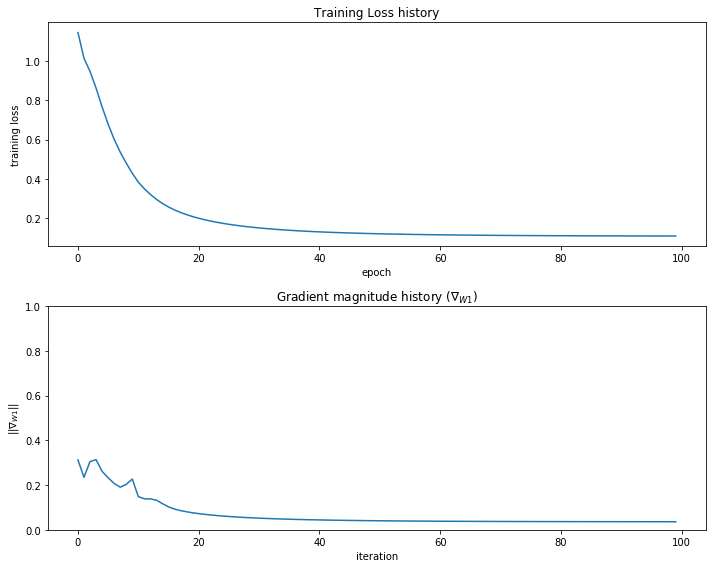### [5pts] Q2.4 Using ReLU activation¶

The Rectified Linear Unit (ReLU) activation is also widely used: $ReLU(x)=max(0,x)$.

• Complete the implementation for the ReLU activation (forward and backward) in mlp.py.
• Train the network with ReLU, and report your final training loss.

Make sure you first pass the numerical gradient check on toy data.

In :
net = init_toy_model('relu', std=1e-1)

loss, grads = net.loss(X, y, reg=0.1)
print('loss = ', loss)  # correct_loss = 1.320973

# The differences should all be very small
f = lambda W: net.loss(X, y, reg=0.1)

loss =  1.3037878913298206
W2 max relative error: 3.440708e-09
b2 max relative error: 3.865091e-11
W1 max relative error: 3.561318e-09
b1 max relative error: 8.994864e-10


Now that it's working, let's train the network. Does the net get stronger learning signals (i.e. gradients) this time? Report your final training loss.

In :
net = init_toy_model('relu', std=1e-1)
stats = net.train(X, y, X, y,
learning_rate=0.1, reg=1e-5,
num_epochs=50, verbose=False)

print('Final training loss: ', stats['loss_history'][-1])

# plot the loss history
fig, (ax1, ax2) = plt.subplots(2, 1)
ax1.plot(stats['loss_history'])
ax1.set_xlabel('epoch')
ax1.set_ylabel('training loss')
ax1.set_title('Training Loss history')
ax2.set_xlabel('iteration')
ax2.set_ylabel(r'$||\nabla_{W1}||$')
ax2.set_title('Gradient magnitude history ' +  r'($\nabla_{W1}$)')
fig.tight_layout()
plt.show()

Final training loss:  0.0178562204869839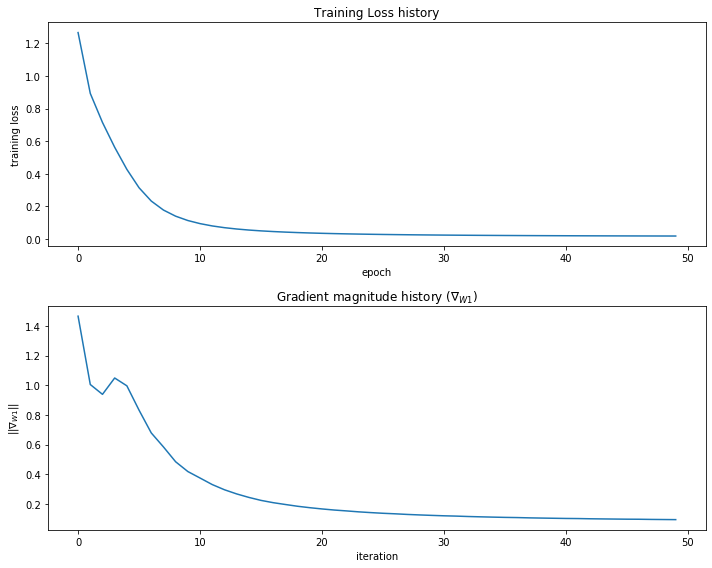## Application to a real Problem¶

Now that you have implemented a two-layer network that works on toy data, let's try some real data. The MNIST dataset is a standard machine learning benchmark. It consists of 70,000 grayscale handwritten digit images, which we split into 50,000 training, 10,000 validation and 10,000 testing. The images are of size 28x28, which are flattened into 784-d vectors.

Note 1: the function get_MNIST_data requires the scikit-learn package. If you previously did anaconda installation to set up your Python environment, you should already have it. Otherwise, you can install it following the instructions here: http://scikit-learn.org/stable/install.html

Note 2: If you encounter a HTTP 500 error, that is likely temporary, just try again.

In :
# load MNIST
from utils import get_MNIST_data
X_train, y_train, X_val, y_val, X_test, y_test = get_MNIST_data()
print('Train data shape: ', X_train.shape)
print('Train labels shape: ', y_train.shape)
print('Validation data shape: ', X_val.shape)
print('Validation labels shape: ', y_val.shape)
print('Test data shape: ', X_test.shape)
print('Test labels shape: ', y_test.shape)

Train data shape:  (50000, 784)
Train labels shape:  (50000,)
Validation data shape:  (10000, 784)
Validation labels shape:  (10000,)
Test data shape:  (10000, 784)
Test labels shape:  (10000,)


### Train a network on MNIST¶

We will now train a network on MNIST with 64 hidden units in the hidden layer. We train it using SGD, and decrease the learning rate with an exponential rate over time; this is achieved by multiplying the learning rate with a constant factor learning_rate_decay (which is less than 1) after each epoch. In effect, we are using a high learning rate initially, which is good for exploring the solution space, and using lower learning rates later to encourage convergence to a local minimum (or saddle point, which may happen more often).

• Train your MNIST network with 2 different activation functions: sigmoid and ReLU.

We first define some variables and utility functions. The plot_stats function plots the histories of gradient magnitude, training loss, and accuracies on the training and validation sets. The show_net_weights function visualizes the weights learned in the first layer of the network. In most neural networks trained on visual data, the first layer weights typically show some visible structure when visualized. Both functions help you to diagnose the training process.

In :
input_size = 28 * 28
hidden_size = 64
num_classes = 10

# Plot the loss function and train / validation accuracies
def plot_stats(stats):
fig, (ax1, ax2, ax3) = plt.subplots(3, 1)
ax1.set_title('Gradient magnitude history ' + r'$(\nabla_{W1})$')
ax1.set_xlabel('Iteration')
ax1.set_ylabel(r'$||\nabla_{W1}||$')

ax2.plot(stats['loss_history'])
ax2.set_title('Loss history')
ax2.set_xlabel('Iteration')
ax2.set_ylabel('Loss')
ax2.set_ylim(0, 100)

ax3.plot(stats['train_acc_history'], label='train')
ax3.plot(stats['val_acc_history'], label='val')
ax3.set_title('Classification accuracy history')
ax3.set_xlabel('Epoch')
ax3.set_ylabel('Clasification accuracy')
fig.tight_layout()
plt.show()

# Visualize the weights of the network
from utils import visualize_grid
def show_net_weights(net):
W1 = net.params['W1']
W1 = W1.T.reshape(-1, 28, 28)
plt.gca().axis('off')
plt.show()


### Sigmoid network¶

In :
sigmoid_net = TwoLayerMLP(input_size, hidden_size, num_classes, activation='sigmoid', std=1e-1)

# Train the network
sigmoid_stats = sigmoid_net.train(X_train, y_train, X_val, y_val,
num_epochs=20, batch_size=100,
learning_rate=1e-3,  learning_rate_decay=0.95,
reg=0.5, verbose=True)

# Predict on the training set
train_acc = (sigmoid_net.predict(X_train) == y_train).mean()
print('Sigmoid final training accuracy: ', train_acc)

# Predict on the validation set
val_acc = (sigmoid_net.predict(X_val) == y_val).mean()
print('Sigmoid final validation accuracy: ', val_acc)

# Predict on the test set
test_acc = (sigmoid_net.predict(X_test) == y_test).mean()
print('Sigmoid test accuracy: ', test_acc)

# show stats and visualizations
plot_stats(sigmoid_stats)
show_net_weights(sigmoid_net)

C:\Users\songd\Downloads\ps3\mlp.py:22: RuntimeWarning: overflow encountered in exp
return 1 / (1 + np.exp(-z))

Epoch 1: loss 79.040004, train_acc 0.160000, val_acc 0.266300
Epoch 2: loss 49.814996, train_acc 0.500000, val_acc 0.461100
Epoch 3: loss 32.419904, train_acc 0.640000, val_acc 0.568300
Epoch 4: loss 21.756599, train_acc 0.630000, val_acc 0.639700
Epoch 5: loss 15.148895, train_acc 0.680000, val_acc 0.685000
Epoch 6: loss 10.909900, train_acc 0.680000, val_acc 0.712600
Epoch 7: loss 8.078106, train_acc 0.760000, val_acc 0.737900
Epoch 8: loss 6.166522, train_acc 0.830000, val_acc 0.755600
Epoch 9: loss 4.948016, train_acc 0.780000, val_acc 0.772900
Epoch 10: loss 4.113118, train_acc 0.760000, val_acc 0.785000
Epoch 11: loss 3.455138, train_acc 0.840000, val_acc 0.797000
Epoch 12: loss 3.026239, train_acc 0.840000, val_acc 0.808100
Epoch 13: loss 2.702231, train_acc 0.840000, val_acc 0.819600
Epoch 14: loss 2.438965, train_acc 0.820000, val_acc 0.830900
Epoch 15: loss 2.258613, train_acc 0.900000, val_acc 0.839900
Epoch 16: loss 2.166625, train_acc 0.860000, val_acc 0.846800
Epoch 17: loss 2.098843, train_acc 0.840000, val_acc 0.852800
Epoch 18: loss 1.975990, train_acc 0.910000, val_acc 0.859300
Epoch 19: loss 1.898398, train_acc 0.900000, val_acc 0.862300
Epoch 20: loss 1.876564, train_acc 0.910000, val_acc 0.866400
Sigmoid final training accuracy:  0.8721
Sigmoid final validation accuracy:  0.8664
Sigmoid test accuracy:  0.8639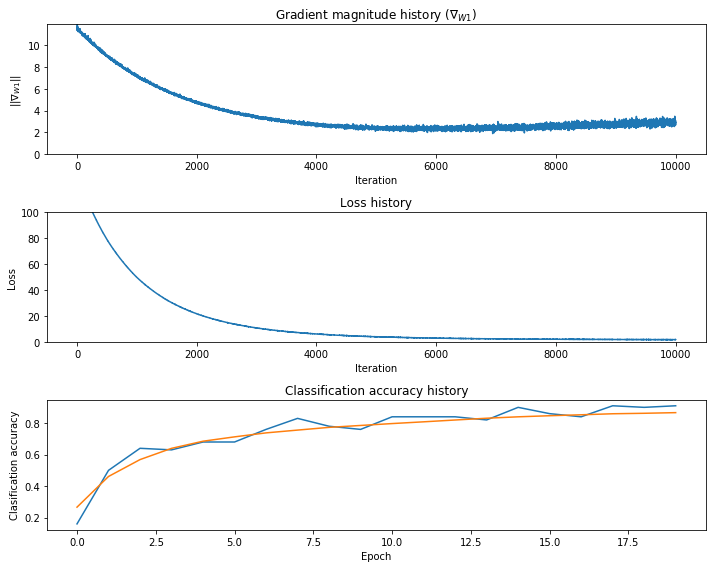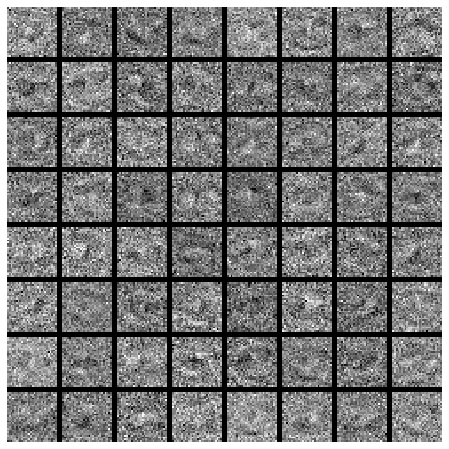### ReLU network¶

In :
relu_net = TwoLayerMLP(input_size, hidden_size, num_classes, activation='relu', std=1e-1)

# Train the network
relu_stats = relu_net.train(X_train, y_train, X_val, y_val,
num_epochs=20, batch_size=100,
learning_rate=1e-3, learning_rate_decay=0.95,
reg=0.5, verbose=True)
# Predict on the training set
train_acc = (relu_net.predict(X_train) == y_train).mean()
print('ReLU final training accuracy: ', train_acc)

# Predict on the validation set
val_acc = (relu_net.predict(X_val) == y_val).mean()
print('ReLU final validation accuracy: ', val_acc)

# Predict on the test set
test_acc = (relu_net.predict(X_test) == y_test).mean()
print('ReLU test accuracy: ', test_acc)

# show stats and visualizations
plot_stats(relu_stats)
show_net_weights(relu_net)

Epoch 1: loss 77.403993, train_acc 0.890000, val_acc 0.871800
Epoch 2: loss 47.584593, train_acc 0.940000, val_acc 0.888800
Epoch 3: loss 29.867657, train_acc 0.950000, val_acc 0.905500
Epoch 4: loss 19.523982, train_acc 0.940000, val_acc 0.921400
Epoch 5: loss 13.036818, train_acc 0.940000, val_acc 0.926900
Epoch 6: loss 8.991179, train_acc 0.930000, val_acc 0.933400
Epoch 7: loss 6.140189, train_acc 0.970000, val_acc 0.941900
Epoch 8: loss 4.527342, train_acc 0.940000, val_acc 0.945300
Epoch 9: loss 3.236795, train_acc 0.980000, val_acc 0.951000
Epoch 10: loss 2.410958, train_acc 0.970000, val_acc 0.954300
Epoch 11: loss 1.849616, train_acc 0.980000, val_acc 0.957000
Epoch 12: loss 1.393771, train_acc 0.990000, val_acc 0.959100
Epoch 13: loss 1.163523, train_acc 0.980000, val_acc 0.958900
Epoch 14: loss 0.993990, train_acc 0.940000, val_acc 0.961200
Epoch 15: loss 0.815937, train_acc 0.970000, val_acc 0.961500
Epoch 16: loss 0.683699, train_acc 0.960000, val_acc 0.962200
Epoch 17: loss 0.625457, train_acc 0.980000, val_acc 0.962500
Epoch 18: loss 0.487950, train_acc 0.990000, val_acc 0.965200
Epoch 19: loss 0.452108, train_acc 0.990000, val_acc 0.965700
Epoch 20: loss 0.404945, train_acc 0.980000, val_acc 0.965900
ReLU final training accuracy:  0.97276
ReLU final validation accuracy:  0.9659
ReLU test accuracy:  0.9653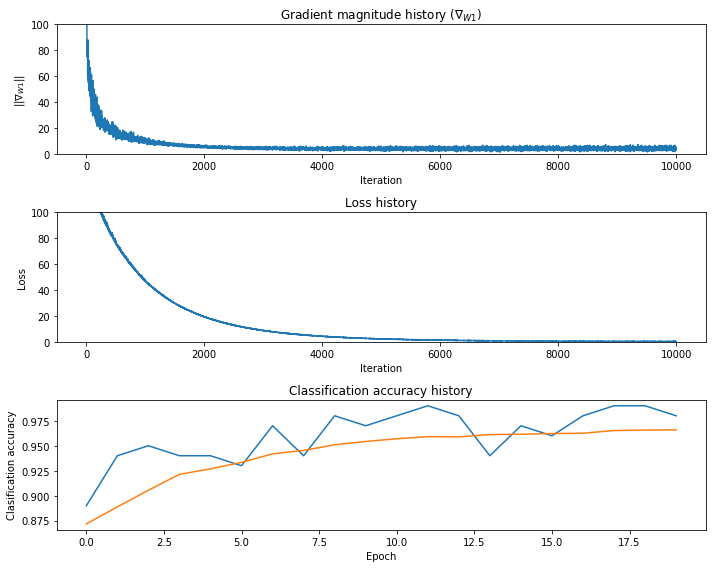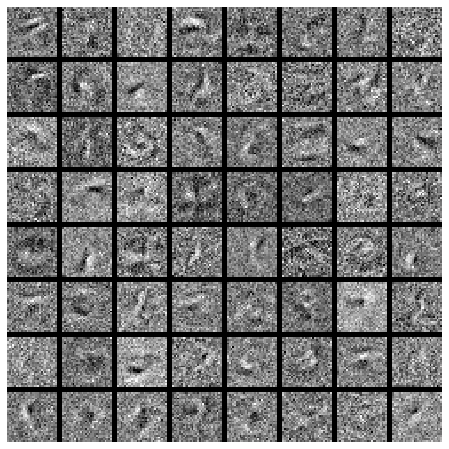### [5pts] Q2.5¶

Which activation function would you choose in practice? Why?

Q2.5 solution: I prefer to use reLU funciton because this funciton doesn't have saturation problem.

## [20pts] Problem 3: Simple Regularization Methods¶

You may have noticed the reg parameter in TwoLayerMLP.loss, controlling "regularization strength". In learning neural networks, aside from minimizing a loss function $\mathcal{L}(\theta)$ with respect to the network parameters $\theta$, we usually explicitly or implicitly add some regularization term to reduce overfitting. A simple and popular regularization strategy is to penalize some norm of $\theta$.

### [10pts] Q3.1: L2 regularization¶

We can penalize the L2 norm of $\theta$: we modify our objective function to be $\mathcal{L}(\theta) + \lambda \|\theta\|^2$ where $\lambda$ is the weight of regularization. We will minimize this objective using gradient descent with step size $\eta$. Derive the update rule: at time $t+1$, express the new parameters $\theta_{t+1}$ in terms of the old parameters $\theta_t$, the gradient $g_t=\frac{\partial \mathcal{L}}{\partial \theta_t}$, $\eta$, and $\lambda$.

Q3.1 solution: ||\theta||^2 = tr(\theta^T \cdot \theta) \\ \frac{||\theta||^2}{\theta} = \frac{\partial tr(\theta^T \cdot \theta)}{\partial \theta} \\ \begin{equation} \begin{aligned} dtr(\theta^T \cdot \theta) &= tr((d\theta^T) \cdot \theta + \theta^Td\theta) \\ &= tr((d\theta^T) \cdot \theta) + tr(\theta^Td\theta) \\ &= tr(2\theta^Td\theta) \\ &= tr((\frac{\partial tr(\theta^T \cdot \theta)}{\partial \theta})^Td\theta) \end{aligned} \end{equation} \\ \frac{\partial tr(\theta^T \cdot \theta)}{\partial \theta} = 2\theta \\ \theta_{t+1} = \theta_t - \eta*(g_t + 2\lambda\theta_t)

### [10pts] Q3.2: L1 regularization¶

Now let's consider L1 regularization: our objective in this case is $\mathcal{L}(\theta) + \lambda \|\theta\|_1$. Derive the update rule.

(Technically this becomes Sub-Gradient Descent since the L1 norm is not differentiable at 0. But practically it is usually not an issue.)

Q3.2 solution $g(x) = x^{\frac{1}{2}} \\ ||\theta||_1 = g(||\theta||_2) d||\theta||_1 = \frac{1}{2} ||\theta||_2^{-\frac{1}{2}} \odot d||\theta_2|| \\ \frac{\partial ||\theta_1||}{\partial \theta} = ||\theta_2||^{-\frac{1}{2}} \odot \theta \\ \theta_{t+1} = \theta_t - \eta*(g_t + \lambda||\theta_2||^{-\frac{1}{2}} \odot \theta)$